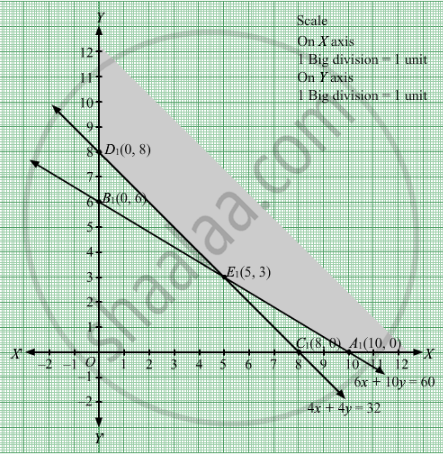# Two Tailors, a and B Earn Rs 15 and Rs 20 per Day Respectively. a Can Stitch 6 Shirts and 4 Pants While B Can Stitch 10 Shirts and 4 Pants per Day. - Mathematics

Sum

Two tailors, A and B earn Rs 15 and Rs 20 per day respectively. A can stitch 6 shirts and 4 pants  while B can stitch 10 shirts and 4 pants per day. How many days shall each work if it is desired to produce (at least) 60 shirts and 32 pants at a minimum labour cost?

#### Solution

Let tailor A work for x days and tailor B work for days.
In one day, A can stitch 6 shirts and 4 pants whereas can stitch 10 shirts and 4 pants
Thus, in  x days A can stitch 6x shirts and 4y pants whereas B can stich 10y shirts and 4y pants.
pants.
It is given that the minimum requirement of the shirts and pants are respectively 60 and 32.
Thus,

$6x + 10y \geq 60$
$4x + 4y \geq 32$

Further it is given that A and B earn Rs 15 and Rs 20 per day respectively.
Thus, A earn Rs 15x and B earn Rs 20y .
Let Z denotes the total cost

$\therefore Z = 15x + 20y$
Days cannot be negative.

∴ $x, y \geq 0$
Min Z =  $15x + 20y$ subject to

$6x + 10y \geq 60$

$4x + 4y \geq 32$

$x, y \geq 0$
First we will convert inequations into equations as follows :
6x + 10y = 60, 4x + 4y = 32, x = 0 and y = 0

Region represented by 6x + 10y ≥ 60:
The line 6x + 10y = 60 meets the coordinate axes at A1(10, 0) and B1(0, 6) respectively. By joining these points we obtain the line6x + 10y = 60. Clearly (0,0) does not satisfies the 6x + 10y = 60. So,the region which does not contains the origin represents the solution set of the inequation 6x + 10y ≥ 60.

Region represented by 4x + 4y ≥ 32:
The line 4x + 4y =32 meets the coordinate axes at C1(8, 0) and D1(0, 8) respectively. By joining these points we obtain the line 4x + 4y = 32.Clearly (0,0) does not satisfies the inequation 4x + 4y ≥ 32. So,the region which does not contains the origin represents the solution set of the inequation 4x + 4y ≥ 32.

Region represented by x ≥ 0 and y ≥ 0:
Since, every point in the first quadrant satisfies these inequations. So, the first quadrant is the region represented by the inequations x ≥ 0, and ≥ 0.
The feasible region determined by the system of constraints 6x + 10y ≥ 60,4x + 4y ≥ 32, x ≥ 0, and y ≥ 0 are as follows.
Thus, the mathematical formulat​ion of the given linear programming problem isThe corner points are D1(0, 8), E1(5, 3) and  A1(10, 0).
The values of Z at these corner points are as follows

 Corner point Z = 15x + 20y D1 160 E1 135 A1 150

The minimum value of Z is 135 which is attained at E1(5, 3).
Thus, for minimum labour cost, A should work for 5 days and B should work for 3 days.
Concept: Graphical Method of Solving Linear Programming Problems
Is there an error in this question or solution?

#### APPEARS IN

RD Sharma Class 12 Maths
Chapter 30 Linear programming
Exercise 30.4 | Q 3 | Page 50